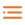# DSA by Google | DSA courses free By Google | Learn DSA free | data structure and algorithm | placement course by google | comitative coding course by google | Google free Courses | google -technilesh.com

Hey readers ,Want to learn the DSA ( data structure and  algorithm ) from beginner to Advanced in any programming language .then ready for it .

The Course content all data structure :

from arrays , link list ,queue to the Binary trees , Graphs and more

## What You Will Learn

#### LESSON 1

1. Introduction and Efficiency
2. Basic introduction to topics covered in this course.
3. Leand the definition of efficiency as well as an explanation of the notation commonly used to describe efficiency.
4. Practice describing efficiency with code snippets.

#### LESSON 2

1. List-Based Collections
2. Learn the formal definition of a list, see definitions and examples of list-based data structures, arrays, linked lists, stacks, and queues.
3. Examine the efficiency of common list methods, and practice using and manipulating these data structures.

#### LESSON 3

1. Searching and Sorting
2. Explore how to search and sort with list-based data structures, including binary search and bubble, merge, and quick sort.
3. Examine the efficiency of each and learn how to use recursion in searching and sorting.
4. See and write examples of these methods, as well as more sorting algorithms like insertion sort.

#### LESSON 4

1. Maps and Hashing
2. Understand the concepts of sets, maps (dictionaries), and hashing.
3. Examine common problems and approaches to hashing, and practice with examples of hash tables and hash maps.

#### LESSON 5

1. Trees
2. Learn the concepts and terminology associated with tree data structures.
3. Investigate common tree types, such as binary search trees, heaps, and self-balancing trees.
4. See examples of common tree traversal techniques, examine the efficiency of traversals and common tree functions, and practice manipulating trees.

#### LESSON 6

1. Graphs
2. Examine the theoretical concept of a graph and understand common graph terms, coded representations, properties, traversals, and paths.
3. Practice manipulating graphs and determining the efficiency associated with graphs.

#### LESSON 7

1. Case Studies in Algorithms
2. Explore famous computer science problems, specifically the Shortest Path Problem, the Knapsack Problem, and the Traveling Salesman Problem.
3. Learn about brute-force, greedy, and dynamic programming solutions to such problems.

### LESSON 8

1. Technical Interviewing Techniques